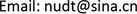1. 引言

2. 数值方法

∂ U ∂ t + ∂ E ∂ x + ∂ F ∂ y + ∂ G ∂ z = ∂ E v ∂ x + ∂ F v ∂ y + ∂ G v ∂ z (1)

3. 动导数计算方法

C m = C m ( α ( t ) , α ˙ ( t ) , α ¨ ( t ) , ⋯ , q ( t ) , q ˙ ( t ) , q ¨ ( t ) , ⋯ ) (2)

α ( t ) = θ = α 0 + α m sin k t (3)

C m θ = k θ m π ∫ t s t s + T c C m ( t ) sin k t d t (4)

C m θ ˙ = 1 θ m π ∫ t s t s + T c C m ( t ) cos k t d t (5)

t s 为积分起始时刻， T c 为振动周期。同样的，强迫俯仰振动时引起的俯仰-滚转力矩交叉耦合导数为：

C m α ˙ + C m q = 1 θ m π ∫ t s t s + T c C l ( t ) cos k t d t (6)

ψ = ψ m sin k t (7)

C l ψ ˙ = C l p + C l β ˙ sin ( α 0 ) = 1 π ψ m ∫ t s t s + T c C l ( t ) cos k t d t (8)

4. 验证算例

5. “十”字翼导弹俯仰阻尼导数计算

“十”字翼导弹的俯仰力矩静、动导数均为负值，说明导弹是俯仰静、动稳定的。随着马赫数的增加，静、动导数的绝对值减小，静、动不稳定性减弱。同时，俯仰–滚转交叉耦合导数为正值，表明在俯仰过程中可能出现滚转方向的动不稳定问题。从表1还可以看出，俯仰–滚转阻尼导数量级很小，比俯仰力矩动导数小4个量级，计算时涉及表面摩阻等复杂的计算问题，增加了计算难度，因此交叉耦合导数计算的正确性和计算精度还需相关实验数据进一步加以验证。

Computation results of dynamic derivatives for missile geometries with fin
MaC m α ˙C m q + C m α ˙C l q + C l α ˙
1.58−44.32−261.400.08001
1.75−37.92−239.990.06054
1.89−33.71−227.850.04700
2.10−29.15−208.900.03174
2.50−23.55−180.490.01749

6. “十”字翼导弹滚转阻尼导数辨识

“十”字翼导弹强迫滚转振动的计算条件为：攻角0˚，侧滑角0˚，飞行高度1 km。马赫数分别为1.53，2.03，2.27，2.54。给定强迫滚转振动形式 ψ = ψ m sin k t ，其中振幅 ψ m 取2.5˚，减缩频率取0.05，质心位置 X c g / L = 0.5 。

Influence of different parameters on the roll damping derivativ
nsubkC l ψ ˙
50.01−13.594
50.05−13.587
50.2−13.339
51−12.282
10.05−13.35
300.05−13.355

7. 结论

1) 本文数值模拟了HBS模型和“十”字翼导弹小振幅强迫振动下的非定常气动力/力矩特性，对强迫运动过程得到的气动力迟滞曲线辨识了俯仰和滚转阻尼导数。通过与实验结果的比较验证了动导数辨识方法的可靠性。此外还开展了减缩频率、子迭代步数、网格规模对动导数辨识结果的影响分析。

2) 超音速条件下“十”字翼导弹俯仰运动时，俯仰–滚转交叉耦合导数有较为明显的周期性规律(但量级很小，与直接阻尼导数相比差4个量级)，而对于俯仰–偏航交叉耦合导数来说，其量值呈不规则的脉动状态变化，与俯仰振动的交叉耦合性很弱。交叉耦合导数计算的正确性还需做进一步的深入研究。